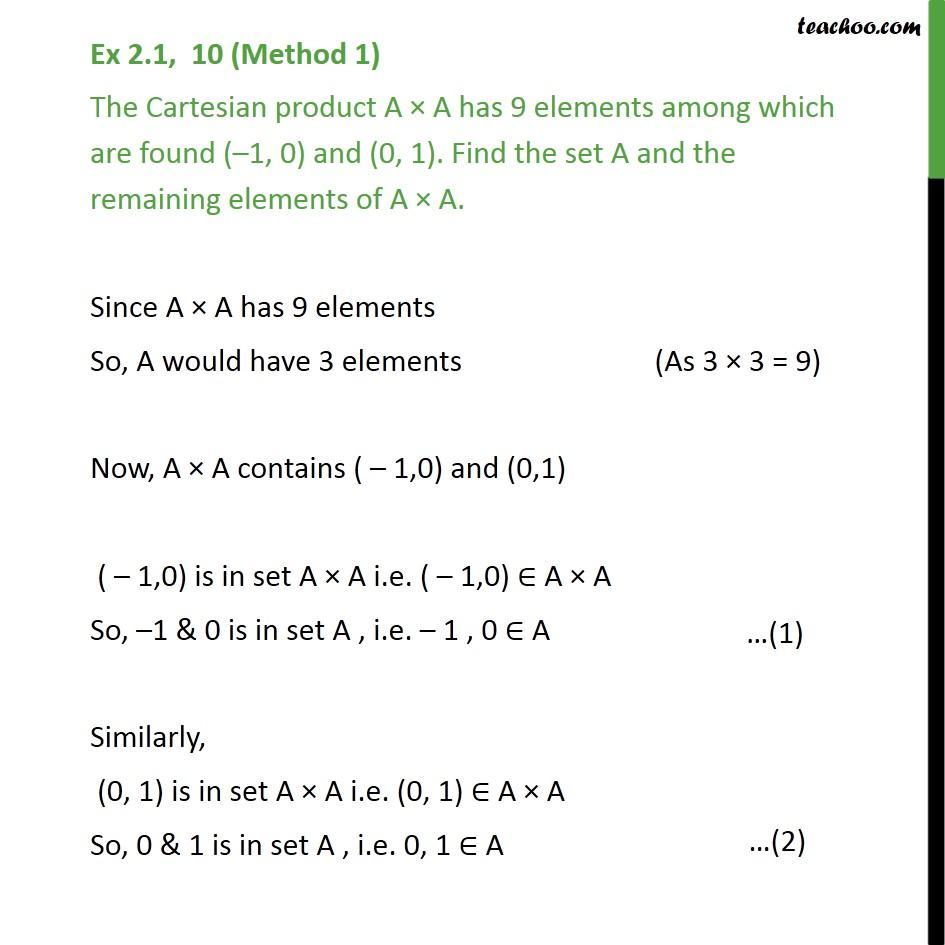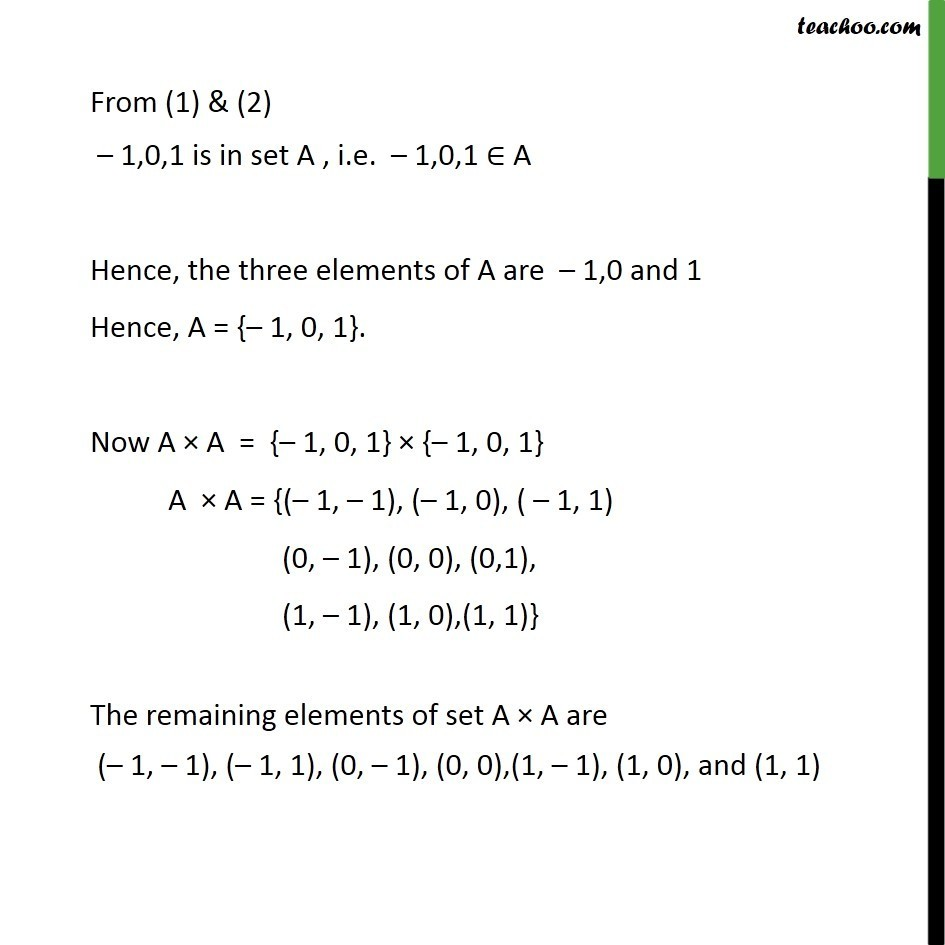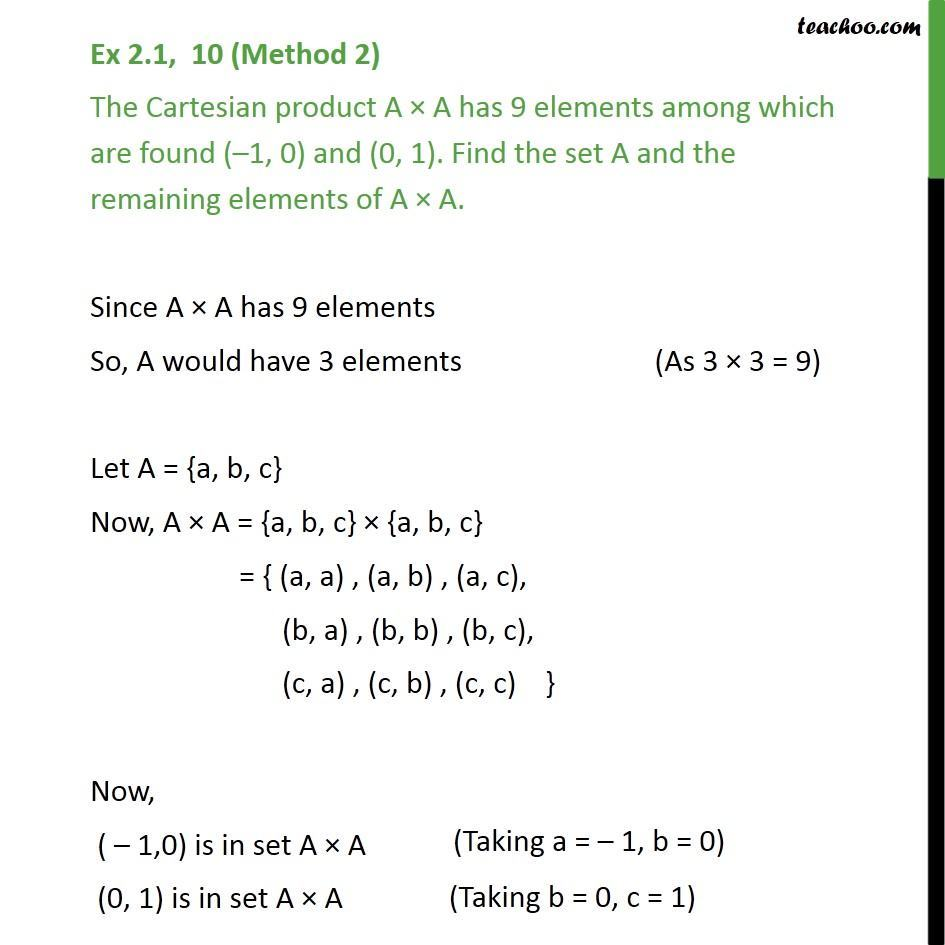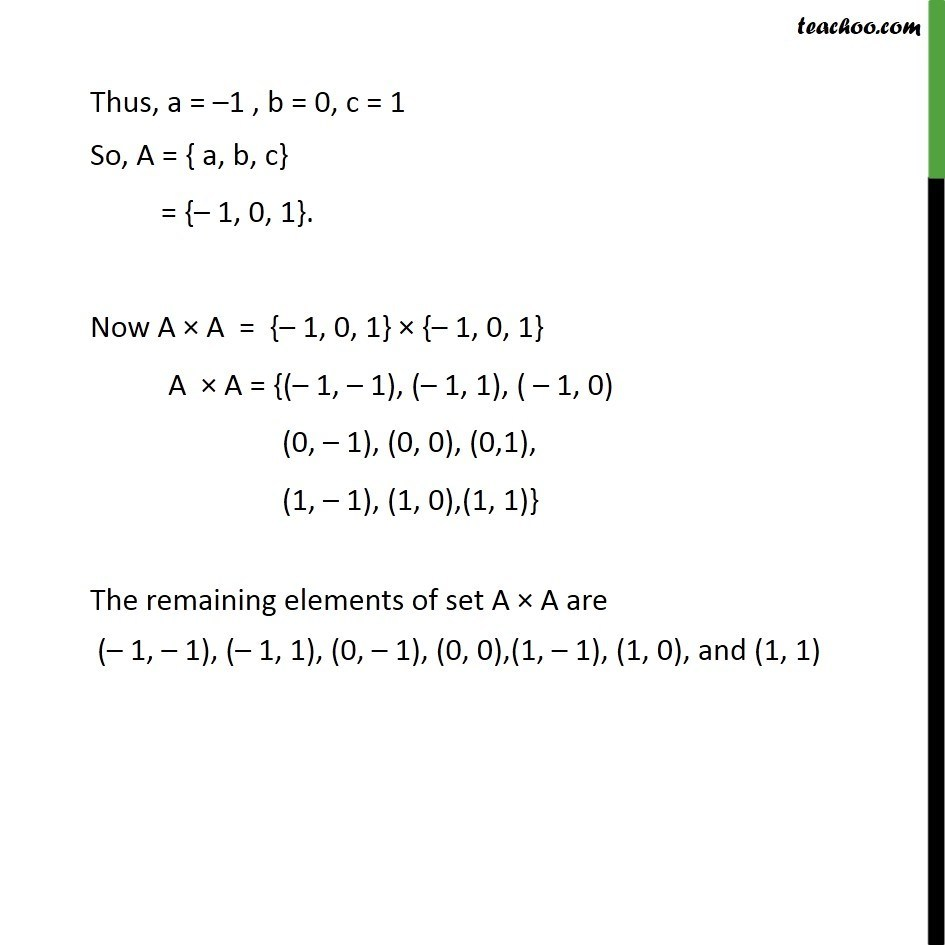1. Chapter 2 Class 11 Relations and Functions
2. Concept wise
3. Number of elements

Transcript

Ex 2.1, 10 (Method 1) The Cartesian product A A has 9 elements among which are found ( 1, 0) and (0, 1). Find the set A and the remaining elements of A A. Since A A has 9 elements So, A would have 3 elements Now, A A contains ( 1,0) and (0,1) ( 1,0) is in set A A i.e. ( 1,0) A A So, 1 & 0 is in set A , i.e. 1 , 0 A Similarly, (0, 1) is in set A A i.e. (0, 1) A A So, 0 & 1 is in set A , i.e. 0, 1 A From (1) & (2) 1,0,1 is in set A , i.e. 1,0,1 A Hence, the three elements of A are 1,0 and 1 Hence, A = { 1, 0, 1}. Now A A = { 1, 0, 1} { 1, 0, 1} A A = {( 1, 1), ( 1, 0), ( 1, 1) (0, 1), (0, 0), (0,1), (1, 1), (1, 0),(1, 1)} The remaining elements of set A A are ( 1, 1), ( 1, 1), (0, 1), (0, 0),(1, 1), (1, 0), and (1, 1) Ex 2.1, 10 (Method 2) The Cartesian product A A has 9 elements among which are found ( 1, 0) and (0, 1). Find the set A and the remaining elements of A A. Since A A has 9 elements So, A would have 3 elements Let A = {a, b, c} Now, A A = {a, b, c} {a, b, c} = { (a, a) , (a, b) , (a, c), (b, a) , (b, b) , (b, c), (c, a) , (c, b) , (c, c) } Now, ( 1,0) is in set A A (0, 1) is in set A A Thus, a = 1 , b = 0, c = 1 So, A = { a, b, c} = { 1, 0, 1}. Now A A = { 1, 0, 1} { 1, 0, 1} A A = {( 1, 1), ( 1, 1), ( 1, 0) (0, 1), (0, 0), (0,1), (1, 1), (1, 0),(1, 1)} The remaining elements of set A A are ( 1, 1), ( 1, 1), (0, 1), (0, 0),(1, 1), (1, 0), and (1, 1)

Number of elements